MORE SITES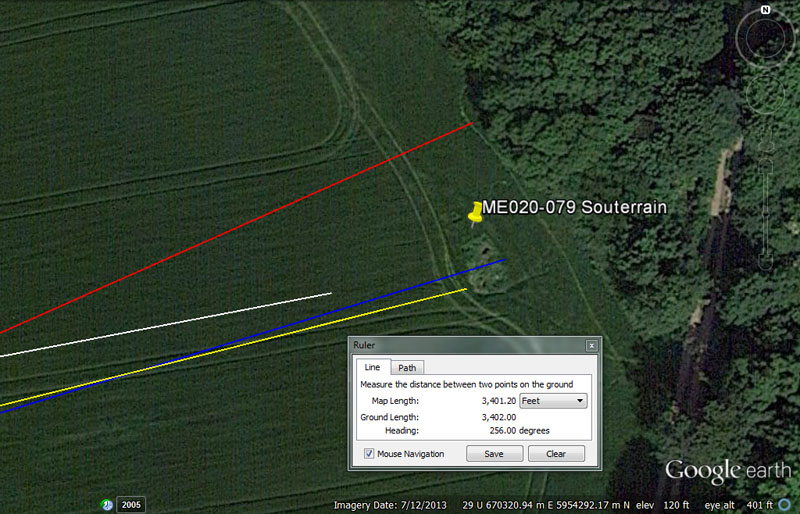This is a large souterrain system that curves in an arc to the NNW and the published reports about it indicate that it was quite extensive.

From Knowth = 15840’ @ 259.2-degrees.

This distance is 3-miles of 5280' each.

The angle relates to the 25920-year Precession of the Equinoxes cycle. A face of the Heel stone at Stonehenge was positioned to sit a coded distance 259.2' from the centre of Stonehenge.

From Newgrange = 13200' or 13333.33333’ @ 66-degrees.

A distance of 13200' would be 2.5-miles of 5280' whereas 13333.3333' would be 1/3rd of 40000'

The 66-degree vector seems to terminate near the northern section of the souterrain system. The sum of 66' would, of course, be 1-chain of length.

From Dowth Mound = 7087.5’ @ 253.125-degrees.

A duration of 7087.5-days would be 20-lunar years of 354.375-days each. The Khafre Pyramid of Egypt has a design length of 708.75'.

The 253.125-degree angle is lunar coding also and there would be 14 periods of 25.3125-days (25 & 5/16ths) in a lunar year.

From Dowth Henge = 3402’ @ 256-degrees .

This distance is lunar coding and there would be 3402-days X 2 in the 6804-day lunar nutation cycle.

The degree angle is navigational coding and reminiscent of dividing the 360-degree circle by half, quarter, eighth, sixteenth, thirty-second, etc. A 256th segment of the 24883.2-mile circumference Earth would be 97.2-miles.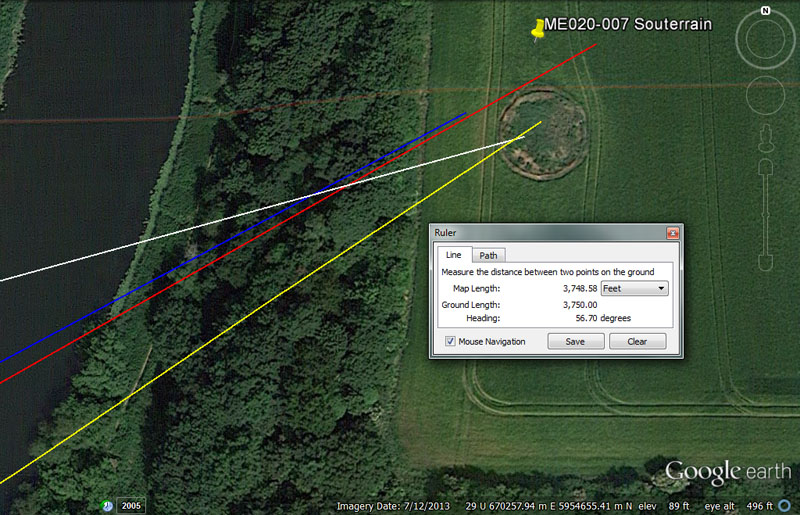This is yet another souterrain system for student accommodation, while out & about on orienteering assignments.

From Knowth = 16000 @ 74.66666-degrees.

A geomancer's mile of 14400 is .9 of 16000'.

The degree angle is navigational and 74.6666' would be 1/90th of an Irish mile of 6720'. It would also be 1/81st of 1-minute of equatorial arc (6048').

From Newgrange = 13750’ @ 61.1111-degrees.

There would be 1.2 increments of 13750' in a league of 16500' (3.125-miles).

There would be 27 increments of 611.1111' (611 & 1/9th) in a league of 16500'.

From Dowth Mound = 7333.33333’ @ 63.36-degrees.

There would be 22.5 increments of 733.33333' in a league of 16500'.

Again, with the angle of 63.36-degrees we see an expression of the Egyptian Royal Cubit @ 20.625". A distance of 6336' (1.2-miles of 5280') would be 1/20625th of the 24750'-mile circumference of the Earth (130680000').

From Dowth Henge = 3750’ - 3780’ @ 56.7-degrees.

The value 3750' would be 5/7ths of a mile of 5250' and 375' would be 1/44th of a league (16500'). Also, 375-miles would be 1/66th of the 24750-mile equatorial circumference of the Earth.

The angle is lunar coding and there would be 12 intervals of 567-days in the 6804-day lunar nutation cycle or 6.25 intervals of 56.7-days in a lunar year, etc.

Orienteers navigating to this souterrain complex could also locate it using a distance code of 3780' (5/8ths of I-minute of arc or 37.5-seconds of arc).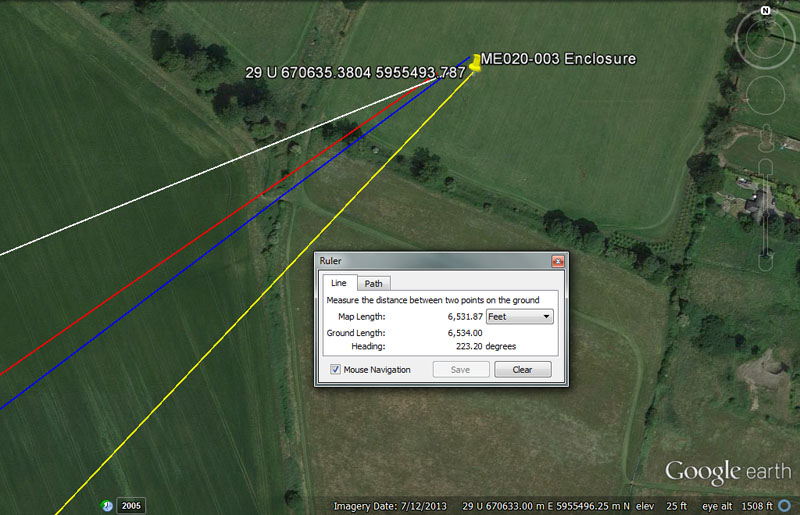Although this enclosure at Oldbridge cannot be visually detected under the field crop in this image, the archaeological record places it at the coordinates shown.

From Knowth = 18144’ or 18150’ @ 247.5-degrees.

This distance is 3-minutes of equatorial arc or 2.7 ancient Irish miles under the 24883.2-mile (of 5250') navigational method. If read as 18150 it would be 3-minutes of arc under the 24750-mile (of 5280') reading.

The degree angle relates to the 24750-mile equatorial circumference.

From Newgrange = 16200’ @ 55-degrees.

The values 1.62, 16.2, 162 or 1620, etc., were very important to ancient calculations and 1.62 represented a rounded form of the PHI ratio of 1.6180339 to one. Numbers based upon rounded forms of both PHI & PI (3.1416) were highly important to ancient calculations and overland distance values were based upon progressions of such numbers.*

The value 16200' would equate to 3 & 1/3rd Roman miles (based upon the 4860' reading). In the 25920-year cycle of the Precession of the equinoxes the sum of 1620-years would be 1/16th part. In the 24883.2-mile equatorial circumference, 16.2-miles would be 1/1536th part or 16200' would be 1/8064th part. In the 6804-day lunar nutation cycle there would be 42 intervals of 162-days, etc.

The angle of 55-degrees is in homage to the line-up of values based upon the number 11, such as the original fathom (merchant fathom) of 5.5'. A rod (16.5') is 3 fathoms, a chain (66') is 12 fathoms, a furlong (660') is 120 fathoms, a mile (5280') is 960 fathoms and a league (16500') is 3000 fathoms.

*Whereas it was absolutely essential for ancient mathematicians and assayers to know the PHI ratio (1.6180339 to 1) in order to square the circle, it was equally important to have a thorough understanding of PI (3.1416 to 1) for navigation.

Sailing ships at sea invariably had to zigzag their way, back & forth across a leyline to a destination. Plotted linear distances or legs of travel at sea (diameters) had to be converted into circumferences at the termination of each leg and it was essential that the scaled circumference number be a nice, whole number that could be easily read in 360 parts. This was done because, at the termination of each leg, the navigator had to calculate the angle back to the point of departure, as well as the angle to the destination and also know exactly where the ship sat in the ocean. (positional plotting).

At Stonehenge a cross-measure on the inside of the slightly elliptical Sarsen Circle achieved 100', therefore producing a circumference of 314.16' (in homage to PI). However, 90-degrees opposed to that cross-measure the internal diameter was 100.8' (1-second of arc in the equatorial circumference of the Earth).

However, from the earliest times we have been able to detect an increment of 315' on sites or as a numerical value used in ancient weights, measures and volumes and, at a late epoch a length of 315' became known as half a Greek stadia or stadium (630' per stadia). The ancient Hebrew Jerusalem Weight standard had a shekel of 315 grains, etc.

If the 100.8' diameter of Stonehenge's inner Sarsen Circle is multiplied by 3.125 (3 & 1/8th), then the result is a circumference value of 315'. We can see from this that seconds or minutes of arc of travel convert to easily read, whole number circumferences that are divisible by 360-degrees and that very reasonable accuracy is achieved using PI at 3.125.

So, in a 315' circle, each 10.5" would be 1-degree of arc. Alternatively, if a navigator was voyaging using minutes of arc for legs of travel, then 6048' X 3.125 = 18900' of circumference or 52.5' per degree (1/10th of a short stadia of 525' or 1/100th of the 5250'-mile).

Certainly, for short voyages around, say, the Mediterranean PI @ 3.125, for easy positional plotting, would have been accurate enough.

Some other examples of PI @ 3.125:

If doing legs in furlongs: 660' X 3.125 = 2062.5 (1200 Egyptian Royal Cubits of circumference or 24750"). Under this reading, every 68.75" would be 1-degree of arc, just like 68.75-miles equating to 1-degree of arc under the 24750-mile navigational system.

If doing legs in miles of 5280': This mile X 3.125 = 16500' (1-league). Under this reading, each 550-inches would be 1-degree of arc.

If doing legs in Irish miles of 6720': This mile X 3.125 = 21000' (4-miles of 5250'). Under this reading each 700" would be 1-degree of arc.

If doing legs in Geomancer's miles of 14400': This mile X 3.125 = 45000' or 125' per degree of arc.

This rounded rendition of PI was loosely used to describe the diameter of the Earth and its circumference under the 5280'-mile reading. Therefore 7920-miles (diameter) X 3.125 = 24750-miles (circumference).

The more accurate renditions of PI used in long voyages:

For traveling much greater distances across "Oceanus" (the Atlantic and other oceans beyond the Mediterranean) navigators would have employed more refined renditions of PI, so that accruing error was minimised.

For navigators calculating legs in increments of the 5250' mile, yet needing a perfect reading on the circumference produced, the version of PI used was 22/7ths (3.142857143). Therefore, 5250' X 22/7ths = 16500' (1-league) where each degree of arc is 550".

For navigators calculating legs in Irish mile increments of 6720' the 22/7ths version of PI was also used. Therefore: 6720' X 22/7ths = 21120' or 704" per degree of arc (58 & 2/3rds feet).

For navigators using minutes of arc of 6048', again 22/7ths was used. Therefore: 6048 X 22/7ths = 19008' or 52.8' per degree of arc.

The special rendition of PI for sea miles of 5940' or 6050', leagues of 16500'and related increments.

Slightly elliptical Silbury Hill in southern England provides us with two diameters in its cross measures. The hill measures 525' (short stadia) in diameter one way and 90-degrees opposed is 550' (1/30th of a league of 16500' ...3.125-miles of 5280').

Montfortescue Hill near the Boyne River Valley of Ireland seems to demonstrate the same cross measure codes as Silbury Hill.

From the Silbury and Montfortescue encoding we can deduce that the 550' diameter was to produce a whole number circumference of 1728' and that the rendition of PI for miles, based upon that "11" family of numbers, was 1728/550ths or 3.141818182.

For navigators completing legs in ancient Scottish miles of 5940': Therefore, 5940 X 1728/550ths = 18662.4 or 51.84' per degree of arc.

For navigators completing legs of travel in minutes of arc of 6050': Therefore, 6050' X 1728/550ths = 19008' or 52.8' per degree of arc.

For navigators completing legs in leagues of 16500': Therefore, 16500' X 1728/550ths = 51840" or 144' per degree of arc.

This same rendition of PI could be used to accurately describe the diameter of the Earth as opposed to its circumference to within 18.8-miles. Therefore, 7920 miles X 1728/550ths = 24883.2-miles.

Looking at the Roman mile (4860) and minute of arc (6075' ... which was also their nautical mile) it seems apparent that they would have used PI @ 3.2.

Therefore, 1-minute of arc X 3.2 = 19440' circumference (20,000 Roman feet of 11.664"). When this is divided by 360-degrees the result is either 54' of 12" or 55 & 5/9ths Roman feet per degree of arc.

Beyond all of the choices the navigator had for completing zigzag legs across the featureless ocean and staying abreast of the ship's position with precision, he required a plotting table, plotting chart or slate, calipers (dividers) and a precisely scaled rule.

From Dowth Mound = 9953.28’ @ 52,8-degrees.

This distance is the same as that which runs from the centre of Dowth Henge to the centre of Newgrange chambered mound. In the equatorial circumference of the Earth the sum of 9953.28-miles X 2.5 = 24883.2. The sum of 995328' would be 1/131.25th of the equatorial circumference.

The degree angle relates to the 5280' mile.

From Dowth Henge = 6534’ @ 43.2-degrees.

The value 6534' is coding related to the equatorial circumference under the 24750-mile (of 5280') navigational method and 6534' would be 1/20000th of the Earth's circumference under that assignment.

The value 432, as found in the degree angle, was very important to ancient lunar and navigational calculations. The length of the Great Pyramid of Egypt (756' of 12") also equates to 432 Hebrew or Celtic cubits of 21". There would be 15.75 intervals of 432-days in the 6804-day lunar nutation cycle. There would be 60 intervals of 432-years in the 25920-year cycle of Precession, etc.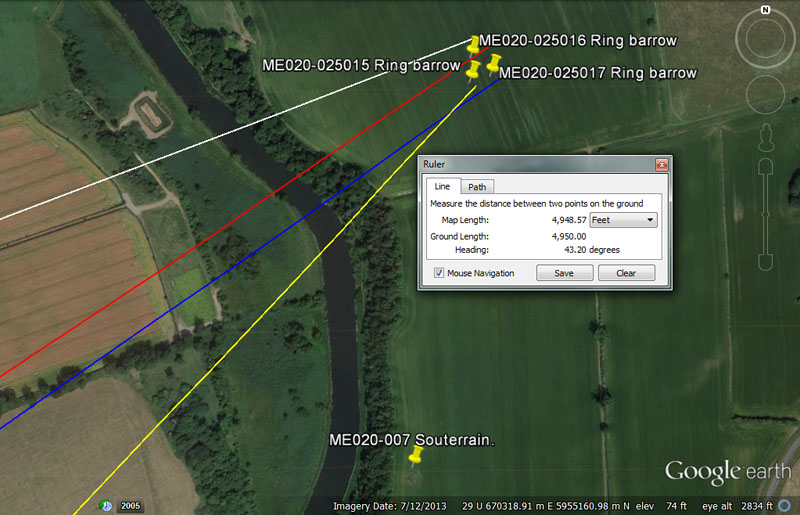A close proximity cluster of 3 ring barrows sit at the positions shown.

From Knowth = 16800 @ 69.12-degrees.

The sum of 16800' would be 3.2-miles or 1/7776th of the equatorial circumference (note: the sum of 777.6-miles would be 1/32nd of 24883.2-miles).

The degree angle relates to 1-degree of arc. Therefore, 24883.2-miles ÷ 360-degrees = 69.12-miles

From Newgrange = 14850’ @ 56-degrees.

This is 2.5 Scottish miles of 5940'.

The degree angle accentuates the important value 56, which was very useful in lunar-cycle calculations, such as: 6804-days ÷ 56 = 121.5-days. Of course the Aubrey Circle at Stonehenge was composed of 56-posts for counts such as this.

From Dowth Mound = 8505 @ 55-degrees.

The distance is in homage to the duration of the 6804-day lunar nutation cycle, of which 850.5 days would be 1/4th. Also, 8505' would be 1.62-miles of 5250'.

The angle of 55-degrees accentuates the ancient fathom of 5.5' and all related increments built upon it (i.e.) rod/perch (3), chain (12), furlong/furrowlong (120), mile (960), league (3000).

From Dowth Henge = 4950’ @ 43.2-degrees.

The sum of 4950-miles would be 1/5th of the 24750-mile equatorial circumference.

The angle accentuates the very important value, 432 used copiously in ancient calculations and 432' would be 1/14th of 1-minute pf equatorial arc (6048'), etc.

There would be several other codes of position in the placement of these 3-sites, as well as tutorials related to the diameters and circumferences of the 3 rings themselves.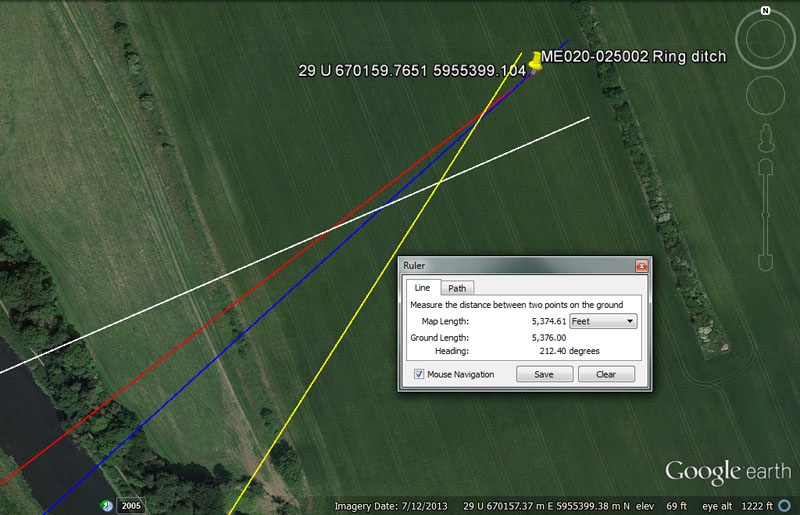The archaeological record places a ring ditch at these coordinates.

From Knowth = 16666.6666 @ 66.6666-degrees.

This distance is 1/3rd of 50000'. In a 3,4,5 triangle the hypotenuse will be 1.6666 greater than one side.

The angle of 66.6666 is 1/3rd of 200. With the invasion of intolerant forms of Christianity into Europe and the British Isles the ages-old beliefs and sciences of the pagani or paysans (peasants and common people) came under assault by the church, which ruthlessly tried to eradicate them. Amongst many other things, the popular little sator-god, Puck (flute-playing herder of flocks, with the lower torso of a goat and bearing horns) was turned into the devil and the 666 numerical combination became the devil's number.

From Newgrange = 14850’ @ 52.5-degrees.

Again, 2.5 Scottish miles.

The angle is in homage to the 5250'-mile.

From Dowth Mound = 8640’ @ 48-degrees.

The sum of 8640-years would be 1/3rd of the 25920-year Precession of the equinoxes cycle and 8640' would be 1/7th of 10-minutes of equatorial arc (60480'). The sun is 864,000-miles wide.

From Dowth Henge = 5376’ @ 32.4-degrees.

This length is 1/24300th of the equatorial circumference and 5376' would be .8 of an ancient Irish mile of 6720'. This length X 1 & 1/8th would be 6048' or 1-minute of equatorial arc.

The degree angle is in homage to a multi-use value (324) much used in ancient calculations. The 25920-year cycle of Precession is 80 X 324-years. The 6804-day lunar nutation cycle is 21 X 324-days. A distance of 32.4-miles would be 1/768th of the 24883.2-mile circumference.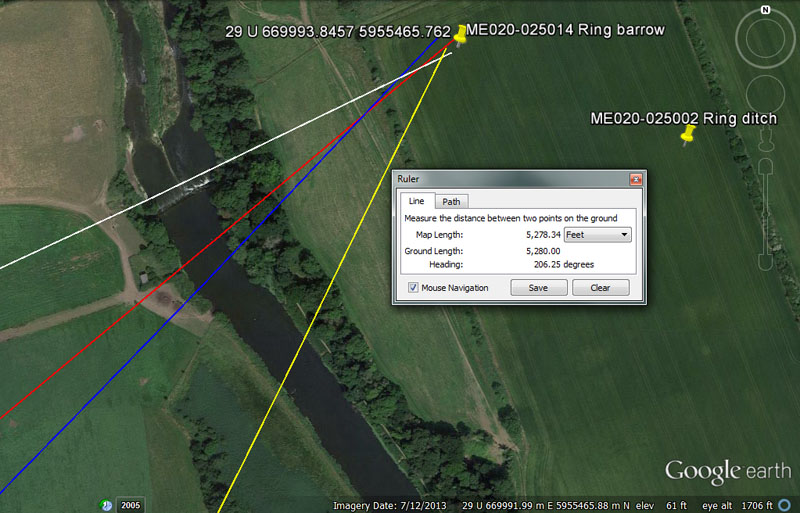Again, the archaeological record shows yet another ring ditch in the top left-hand corner of this Oldbridge field.

From Knowth = 16200’ @ 64.8-degrees.

In the 25920-year cycle of Precession there would be 16 intervals of 1620-years. There would be 42 intervals of 162-days in the 6804-day lunar nutation cycle. The value 1.62 is rounded PHI and, by its inclusion, distances and durations could be read in pseudo-PHI increments. The 16200' distance could be read as 3 & 1/3rd Roman miles.

The degree angle also relates to the cycle of Precession and 648-years would be 1/40th of 25920-years. Likewise, 64.8-miles would be 1/384th of the 24883.2-mile circumference. In the 6804-day lunar nutation cycle, there would be 10.5 intervals of 648-days, etc.

From Newgrange = 14580’ @ 230.4- (50.4) return.

This distance is 3 Roman miles (using the 4860' value).

The degree angle relates to the equatorial circumference of the Earth and 230.4-miles would be 1/108th of 24883.2-miles. Likewise, there would be 230.4 lunar months in the 6804-day lunar nutation cycle. A lunar month is 29.53125-days (29 & 17/32nds ... this value being accurate to within 1 minute of the actual duration). In the 25920-year Precession of the Equinoxes cycle 11.25 X 2304-years = 1 complete cycle.

The return angle of 50.4-degrees is navigational coding and 50.4' would be 1/2 of 1-second of equatorial arc (100.8').

From Dowth Mound = 8333.33333’ @ 64 - 224-degrees.

This distance is 1/3rd of 25000'.

Again the degree angle (64 ... 8 X 8) was a very versatile value for many calculations. The 180-degrees opposed angle (224) was likewise highly useful and 2240 1bs (20 CWT of 112 lbs each) became the ton weight.

From Dowth Henge = 5280’ @ 206.25-degrees.

This distance is, of course, 1-mile.

The angle relates to the mile reading and 3072 Egyptian Royal cubits of 20.625" (20 & 5/8ths) = 5280'. Likewise the sum of 2062.5-miles would be 1/12th of the 24750-mile equatorial circumference.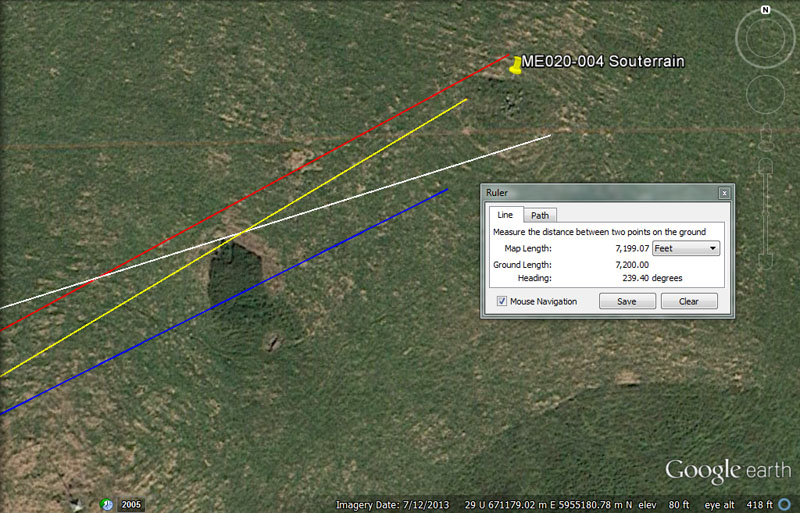More souterrain accommodation could be found at this position.

From Knowth = 19440’ @ 72.6-degrees.

The length of 19440' would be 4 Roman miles using the 4860' reading. Likewise, the sum of 194.4-miles would be 1/128th of the 24883.2-mile equatorial circumference. Also, 19440' would be 1/6720th of the 130636800' (24883.2-mile) circumference using the mile of 5250'. In other words, there would be 19440 ancient Irish miles in the equatorial circumference under this navigational method.

There would be 35 intervals of 194.4-days in the 6804-day lunar nutation cycle or 13.3333 X 1944-years in the 25920-year duration of Precession.

The degree angle is navigational coding related to the 24750-mile (of 5280') equatorial circumference and 7260' would be 1/50th of 1-degree of equatorial arc (363000'). Alternatively, 7260' would be 1/18000th of the 24750-miles (130680000') circumference.

From Newgrange = 17187.5’ @ 61.44-degrees.

The distance is navigational coding and 171.875-miles (171 & 7/8ths) would be 1/144th of the 24750-mile circumference or 2.5-degrees of arc. The distance of 17187.5' would be 10,000 Egyptian Royal cubits of 20.625" each.

The degree angle is navigational coding and the sum of 61.44-miles would be 1/405th of the 24883.2-mile equatorial circumference.

From Dowth = 10800’ @ 63.36-degrees.

This value is multi-use and can be applied to many types of calculations. there would be 108' X 56 in 1-minute of equatorial arc (6048'). There would be 240 X 108-years in the 25920-year Precessional cycle. There would be 63 intervals of 108-days in the 6804-day lunar nutation cycle, etc.

The degree angle coding is navigational and a distance of 6336' (1.2-miles of 5280') would be 1/20625th of the 24750'-mile circumference of the Earth (130680000'). In other words this related to reading the equatorial circumference in Egyptian royal cubits, of which there were 3 types (20.61818", 20.625" & 20.736"), all identified by William Flinders Petrie in his 19th century measurements at Giza.

From Dowth Henge = 7200’ @ 59.4-degrees.

This distance is 1/2 of a geomancer's mile (14400').

The degree angle relates to the mile of 5940'.

Continue